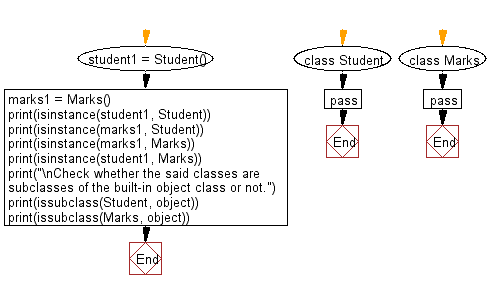﻿ Python: Check instances and in subclasses a given class - w3resource# Python: Check instances and in subclasses a given class

## Python Class ( Basic ): Exercise-8 with Solution

Write a Python program to crate two empty classes, Student and Marks. Now create some instances and check whether they are instances of the said classes or not. Also, check whether the said classes are subclasses of the built-in object class or not.

Sample Solution:

Python Code:

``````class Student:
pass
class Marks:
pass
student1 = Student()
marks1 = Marks()
print(isinstance(student1, Student))
print(isinstance(marks1, Student))
print(isinstance(marks1, Marks))
print(isinstance(student1, Marks))
print("\nCheck whether the said classes are subclasses of the built-in object class or not.")
print(issubclass(Student, object))
print(issubclass(Marks, object))
```
```

Sample Output:

```True
False
True
False

Check whether the said classes are subclasses of the built-in object class or not.
True
True
```

Flowchart:## Visualize Python code execution:

The following tool visualize what the computer is doing step-by-step as it executes the said program:

Python Code Editor:

What is the difficulty level of this exercise?

Test your Programming skills with w3resource's quiz.

﻿

## Python: Tips of the Day

The Zip() Function:

```>>> students = ('John', 'Mary', 'Mike')
>>> ages = (15, 17, 16)
>>> scores = (90, 88, 82, 17, 14)
>>> for student, age, score in zip(students, ages, scores):
...     print(f'{student}, age: {age}, score: {score}')
...
John, age: 15, score: 90
Mary, age: 17, score: 88
Mike, age: 16, score: 82
>>> zipped = zip(students, ages, scores)
>>> a, b, c = zip(*zipped)
>>> print(b)
(15, 17, 16)
```